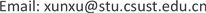1. 引言

2. 问题描述及模型分析

G F i , M o n i t o r = ∪ j = 1 ∞ { ( x , y , z ) | ( x − x i , 0 ) 2 + ( y − y i , 0 ) 2 ≤ ( z i , 0 − z r o a d Δ h 0 r 0 ) 2 , ( x i , j , y i , j , z i , j ) ∈ F i } .

G C = G g e n e r a l − G = ∪ ( x R o a d , y R o a d , z R o a d ) ∉ G R o a d { ( x , y , z ) | ( x − x R o a d ) 2 + ( y − y R o a d ) 2 ≤ ( z − z R o a d Δ h 0 r 0 ) 2 } ≠ ∅

3. 近似算法研究

STEP1：对区域Ggeneral使用栅格法进行空间的划分，以道路所在单元中心为圆心画圆确定G，将 G 1 = G 所在的单元视为LSCP问题中的候选拍摄点，建立整数规划模型。使用分支定界法求解，并确定 circle_min。

STEP2：检查STEP1中求出的 c i r c l e _ min 个拍摄点，若存在两点之间的距离小于 Δ s = v U A V t ，( v U A V 为无人机的速度， Δ s 为无人机拍摄两张照片所需的最小飞行间距，在一般情况下，如果 L i , L i + 1 两点间的距离小于 Δ s ，可以降低无人机的速度，使得无人机从 L i 飞到时，相机能够拍照，但是降低速度无疑会使完成任务的时间变长，而灾害救援系统有时间限制)，则从 G 1 删去处于更“稠密”区域的那点。返回到STEP1重新计算。如果所有点的距离均不小于 Δ s ，则计算当前方案的总权重，执行STEP3。

STEP3：使用启发式算法求解出满足预定精度的方案或使用遍历算法得到最优方案。

STEP4：求解出访问STEP3中求出的最优方案中 c i r c l e _ min 个单元的先后顺序。

4. 算例研究

5. 结束语